# Problems on Numbers - Quantitative Aptitude (MCQ) questions

Dear Readers, Welcome to Quantitative Aptitude Problems on Numbers questions and answers with explanation. These Problems on Numbers solved examples with shortcuts and tricks will help you learn and practice for your Placement Test and competitive exams like Bank PO, IBPS PO, SBI PO, RRB PO, RBI Assistant, LIC,SSC, MBA - MAT, XAT, CAT, NMAT, UPSC, NET etc.

After practicing these tricky Problems on Numbers multiple choice questions, you will be exam ready to deal with any objective type questions.

1)   A group wanted to renovate their club. Each member contributed an amount equal to twice the number of members in the club. But the government contributed same amount as the number of members. If each member had contributed the same amount as the number of members and the government had given an amount twice the number of members, then they would have Rs. 210 less. How many members are there?
- Published on 11 Apr 17

a. 7
b. 15
c. 14
d. 24
 Answer  Explanation ANSWER: 15 Explanation: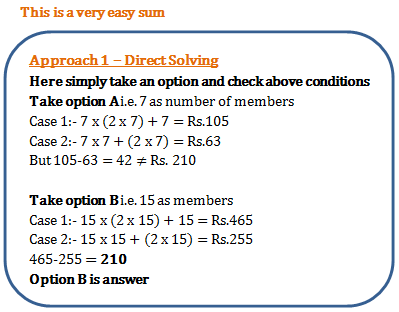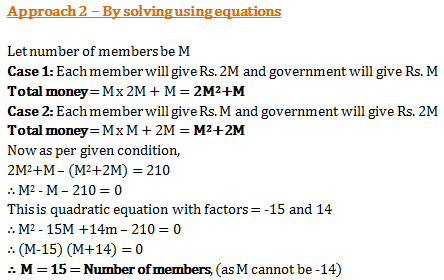2)   ¾ part of tank is full of water. When 30 litres of water is taken out it becomes empty. The capacity of the tank is?
- Published on 05 Apr 17

a. 36 litres
b. 42 litres
c. 40 litres
d. 38 litres
 Answer  Explanation ANSWER: 40 litres Explanation: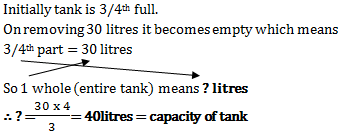3)   The sum of four consecutive integers is 1290. The greatest of them is?
- Published on 05 Apr 17

a. 321
b. 322
c. 323
d. 324
 Answer  Explanation ANSWER: 324 Explanation: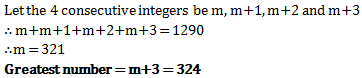4)   280 oranges are divided among some boys and girls whose total number is 50 so that each boy gets 5 oranges and each girl get 7 oranges. The number of girls is
- Published on 05 Apr 17

a. 30
b. 35
c. 15
d. 20
 Answer  Explanation ANSWER: 15 Explanation: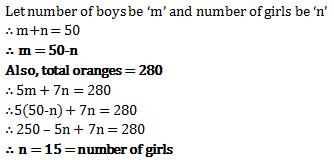5)   A number is multiplied by its one-third to get 192. Find the number.
- Published on 05 Apr 17

a. 16
b. 20
c. 24
d. 28
 Answer  Explanation ANSWER: 24 Explanation: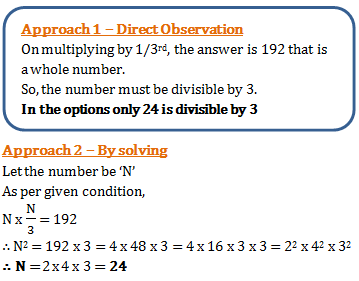6)   A boy was asked to multiply a number by 12. But he multiplied by 21 and got his answer 63 more than the correct answer. The number to be multiplied was
- Published on 05 Apr 17

a. 7
b. 9
c. 8
d. 12
 Answer  Explanation ANSWER: 7 Explanation: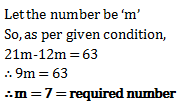7)   On exchanging the digits in unit’s and ten’s place, the difference between original and new numbers becomes 27. The digit in the unit's place is 2 times the digit in the hundred's place. The digit in the ten’s place is 3 times the digit in the hundred’s place. What is 75% of the original number?
- Published on 27 Mar 17

a. 99
b. 369
c. 297
d. 396
 Answer  Explanation ANSWER: 297 Explanation: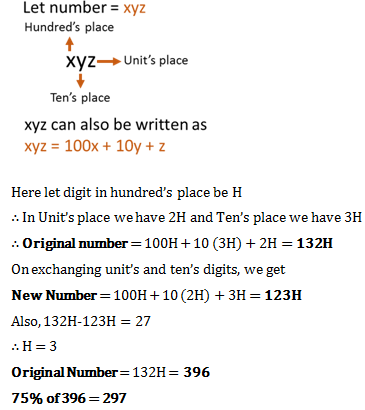8)   The sum of 2 numbers is 36 and their product is 248. What will be the sum of their reciprocals?
- Published on 27 Mar 17

a. 9/62
b. 65/248
c. 212
d. 248/36
 Answer  Explanation ANSWER: 9/62 Explanation: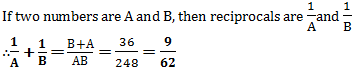9)   Raman has 2 urns. Both these urns have some pebbles. If 20 pebbles from urn B are shifted to urn A then, the number of pebbles in each urn become interchanged. But if 10 pebbles from urn A are put into urn B, then number of pebbles in B are twice the number in A. How many pebbles do A and B have respectively?
- Published on 27 Mar 17

a. 60 and 40
b. 80 and 100
c. 70 and 90
d. 50 and 70
 Answer  Explanation ANSWER: 50 and 70 Explanation: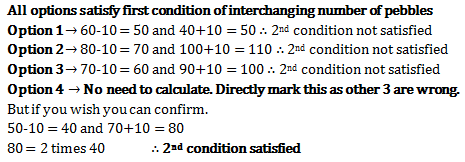10)   Average of 3 numbers is 87. The 1st number is 4 times the 2nd number and 5 times the 3rd number. What will be difference between first and third number?
- Published on 27 Mar 17

a. 99
b. 144
c. 132
d. 169
 Answer  Explanation ANSWER: 144 Explanation: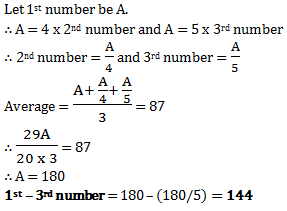1 2 3 4 5 6